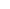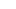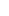###### Forex Trade Results July 26, 2022 – \$1,640
July 26, 2022July 28, 2022

# How to Calculate Margin Level.

## Example #1: Open a long USD/JPY position with 1 mini lot

Let’s say you have an account balance of \$1,000.### Step 1: Calculate Required Margin

You want to go long USD/JPY and want to open 1 mini lot (10,000 units) position. The Margin Requirement is 4%.

How much margin (Required Margin) will you need to open the position?

Since USD is the base currency. this mini lot is 10,000 dollars, which means the position’s Notional Value is \$10,000.

`Required Margin = Notional Value x Margin Requirement \$400 = \$10,000 x .04`

Assuming your trading account is denominated in USD, since the Margin Requirement is 4%, the Required Margin will be \$400.### Step 2: Calculate Used Margin

Aside from the trade we just entered, there aren’t any other trades open.

Since we just have a single position open, the Used Margin will be the same as Required Margin.### Step 3: Calculate Equity

Let’s assume that the price has moved slightly in your favor and your position is now trading at breakeven.

This means that your Floating P/L is \$0.

Let’s calculate the Equity:

`Equity = Account Balance + Floating Profits (or Losses) \$1,000 = \$1,000 + \$0`

The Equity in your account is now \$1,000.### Step 4: Calculate Margin Level

Now that we know the Equity, we can now calculate the Margin Level:

`Margin Level = (Equity / Used Margin) x 100% 250% = (\$1,000 / \$400) x 100%`

The Margin Level is 250%.If the Margin Level is 100% or less, most trading platforms will not allow you to open new trades.

In the example, since your current Margin Level is 250%, which is way above 100%, you’ll still be able to open new trades.

Imagine the Margin Level as being a traffic light.

As long as the Margin Level is above 100%, then your account has the “green light” to continue to open new trades.## Learn to Currency Trade Forex

If you’d like to earn extra income trading on the Forex market, consider learning how to currency trade with Forex Smart Trade.   With their super-accurate proprietary trading tools and best-in-the-business, personalized one-on-one training, you’ll be successful.  Check out the Forex Smart Trade webinar.  It shows one of their trader’s trading and how easy, intuitive, and accurate the tools are.  Or try the Forex Smart Trade 14-day introductory trial for just TEN dollars.##### Paul McMann